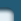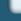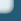Approximation

Web design by Alexei Kuntsevich## Selected Publications

### Keyword(s): approximation


F. Kappel and W. Schappacher. Approximation of functional differential equations by finite dimensional systems. In R. Conti, G. Sestini, and G. Villari, editors, Equazioni differenziali ordinarie ed equazioni funzionali, Proc. Equa-Diff 78, Florence, May 24–30, 1978, pages 479–484, 1978.


F. Kappel and W. Schappacher. Autonomous nonlinear functional differential equations and averaging approximations. Nonlinear Analysis: TMA, 2:391–422, 1978.


F. Kappel. Approximation of functional-differential equations by ordinary differential equations and hereditary control problems. In J. Stoer, editor, Optimization Techniques, Vol. I, Proc. 8th IFIP Conf. Optimization Techniques, Würzburg, September 5–9, 1977, volume 6 of Lecture Notes in Control and Information Sciences, pages 103–108, Berlin, 1978. Springer Verlag.


H. T. Banks and F. Kappel. Spline approximations for functional differential equations. J. Differential Eqs., 34:496–522, 1979.


F. Kappel and K. Kunisch. Spline approximations for neutral functional differential equations. SIAM J. Numerical Analysis, 18:1058–1080, 1981.


F. Kappel. Approximation of neutral functional differential equations in the state space Rn times Lp. In M. Farkas, editor, Qualitative Theory of Differential Equations, Vol. I, Colloquia Mathematica Societatis Janos Bolyai, Vol. 30, pages 463–506. Janos Bolyai Math. Soc. and North Holland Publ. Comp., 1982.


F. Kappel. An approximation scheme for delay equations. In V. Lakshmikantham, editor, Nonlinear Phenomena in Mathematical Sciences, Proc. Internat. Conf. Nonl. Phenomena Math. Sci., Arlington (Texas), June 16–20, 1980, pages 585–595, New York, 1982. Academic Press.


F. Kappel and K. Kunisch. Approximation of the state of infinite delay and Volterra-type equations. In L. Collatz, G. Meinardus, and W. Wetterling, editors, Differenzen-Differentialgleichungen, Anwendungen und numerische Probleme, Proc. Workshop in Oberwolfach, June 6–12, 1982, pages 149–168, Basel, 1983. Birkhäuser.


F. Kappel, K. Kunisch, and G. Moyschewitz. An approximation scheme for infinite delay equations of Volterra type: Numerical results. Technical Report 11, Institutes for Mathematics–Technical University and University of Graz, 1983.


F. Kappel. Finite dimensional approximation to systems with infinite dimensional state space. In H.-W. Knobloch and K. Schmitt, editors, Equadiff 82, Proc. Equa-Diff 82, Würzburg, August 23–28, 1982, volume 1017, pages 287–299, Berlin, 1983. Springer Verlag.


F. Kappel and G. Propst. Approximation of feedback controls for delay systems using Legendre polynomials. Confer. Mat. Sem. Mat. Univ. Bari, 201:1–36, 1984.


F. Kappel, K. Kunisch, and G. Moyschewitz. An approximation scheme for parameter estimation in infinite delay equations of Volterra type: Numerical results. Technical Report 51, Institutes for Mathematics – Technical University and University of Graz, 1984.


F. Kappel. Galerkin type approximation schemes for delay systems. Annales of Differential Equations, 1:57–82., 1985.


F. Kappel. Spline approximation for autonomous nonlinear functional differential equations. Nonlinear Analysis: TMA, 10:503–513, 1986.


F. Kappel and D. Salamon. Spline approximation for retarded systems and the Riccati equation. SIAM J. Control and Optimization, 25:1082–1117, 1987.


F. Kappel and K. Schmitt. Periodic solutions of systems of ordinary differential equations which approximate delay equations. Differential and Integral Eqs., 1:183–212, 1988.


H. T. Banks and F. Kappel. Transformation semigroups and L1-approximation for size structured population models. Semigroup Forum, 38:141–155, 1989.


K. Ito and F. Kappel. Approximation of infinite delay and Volterra type equations. Numerische Math., 54:405–444, 1989.


F. Kappel and G. Peichl. Preservation of controllability under approximation for delay systems. Matematica Aplicada e Computational, 8:23–47, 1989.

This paper deals with controllability of differential equations with a single delay and its finite dimensional approximations. A set of hypotheses is presented which allow a unified treatment of various approximation schemes. Controllability of the delay equation as well as of the approximating ordinary differential equations is characterized by a finite number of linear homogeneous equations which in both cases are closely related. For the approximating systems the size of the linear system of equations which characterizes controllability is independent of the order of approximation.


F. Kappel and D. Salamon. On the stability properties of spline approximation for retarded systems. SIAM J. Control and Optimization, 27:407–431, 1989.


F. Kappel and D. Salamon. An approximation theorem for the algebraic Riccati equation. SIAM J. Control and Optimization, 28:1136–1147, 1990.

For an infinite dimensional linear quadratic control problem in Hilbert space, approximation of the solution of the algebraic Riccati operator equation in the strong operator topology is considered under conditions weaker than uniform exponential stability of the approximating systems. As an application strong convergence of the approximating Riccati operators in case of a previously developed spline approximation scheme for delay systems is established. Finally we investigate convergence of the transfer-functions of the approximating systems.


K. Ito and F. Kappel. A uniformly differentiable approximation scheme for delay systems using splines. Appl. Math. Optim., 23:217–262, 1991.

A new spline-based scheme is developed for linear retarded functional differential equations within the framework of semigroups on the Hilbert space bold Rn times L2. The approximating semigroups inherit in a uniform way the characterization for differentiable semigroups from the solution semigroup of the delay system (e.g. among other things the logarithmic sectorial property for the spectrum). We prove convergence of the scheme in the state spaces bold Rn times L2 and H1. The uniform differentiability of the approximating semigroups enables us to establish error estimates including quadratic convergence for certain classes of initial data. We also apply the scheme for computing the feedback solutions to linear quadratic optimal control problems.


K. Ito, F. Kappel, and G. Peichl. A fully discretized approximation scheme for size structured population models. SIAM J. Numerical Analysis, 28:923–954, 1991.

An efficient algorithm for computing solutions to a class of models for size structured populations is presented. Furthermore, some numerical examples are discussed.


F. Kappel. Approximation of LQR-problems for delay systems: a survey. In K. Bowers and J. Lund, editors, Computation and Control II, pages 187–224, Boston, 1991. Birkhäuser.


K. Ito and F. Kappel. Two families of approximation schemes for delay systems. Results in Mathematics, 21:93–137, 1992.

Based on the Trotter-Kato approximation theorem for strongly continuous semigroups we develop a general framework for the approximation of delay systems. Using this general framework we construct two families of concrete approximation schemes. Approximation of the state is done by functions which are piecewise polynomials on a mesh (m-th order splines of deficiency m). For the two families we also prove convergence of the adjoint semigroups and uniform exponential stability, properties which are essential for approximation of linear quadratic control problems involving delay systems. The characteristic matrix of the delay system is in both cases approximated by matrices of the same structure but with the exponential function replaced by approximations where Padé fractions in the main diagonal resp. in the diagonal below the main diagonal of the Padé table for the exponential function play an essential role.


F. Kappel and Kangpei Zhang. Approximation of linear age-structured population models using Legendre polynomials. J. Math. Anal. Appl., 180:518–549, 1993.

We develop a numerical algorithm for approximation of solutions for linear age–structured population models. The construction is based on approximation of age distributions by modified Legendre polynomials and uses the Trotter-Kato theorem of semigroup theory for the corresponding abstract Cauchy problem. Unbounded resp. nonintegrable mortality rates are admissible.


K. Ito and F. Kappel. Approximation of semilinear equations. Nonlinear Analysis: Theory, Methods and Applications, 24:51–80, 1995.

In this paper we prove well-posedness and approximation results for semilinear evolution equations in Banach spaces. These results are applicable to nonlinear delay equations with state and time dependent delays. As an example we consider a model in epidemics with threshold effects in the mechanism of infection.


P. Bachhiesl, H. Hutten, F. Kappel, K. Köpke, and H. Scharfetter. Dynamical control of the dialysis process. Part I: Structural considerations and first mathematical approach. Biomedizinische Technik, 41:196–202, 1996.


P. Bachhiesl, H. Hutten, F. Kappel, and H. Scharfetter. Dynamical control of the dialysis process. Part II: An improved algorithm for the solution of a tracking problem. Biomedizinische Technik, 41:228–235, 1996.


P. Bachhiesl, H. Hutten, F. Kappel, and H. Scharfetter. Dynamische Kontrolle von Dialyseparametern: ein effizienter Algorithmus zur Lösung eines Tracking Problems. Biomedizinische Technik, 41, Ergänzungsband 1:86–87, 1996.


F. Kappel. The Trotter-Kato theorem and approximation of abstract Cauchy problems. In Bambang Soedijono Subanar and Sri Wahyuni, editors, Proceedings of the Mathematical Analysis and Statistics Conference, Yogyakarta 1995, Part A: Theory and Methods, pages 50–58, 1996. ISBN 979-95118-0-1.


K. Ito and F. Kappel. The Trotter-Kato theorem and approximation of PDEs. Math. Computation, 67:21–44, 1998.

We present formulations of the Trotter-Kato theorem for approximation of linear C0-semigroups which provide very useful framework when convergence of numerical approximations to solutions of PDEs are studied. Applicability of our results is demonstrated using a first order hyperbolic equation, a wave equation and Stokes' equation as illustrative examples.


Abd El Rahman Aly Hussein and F. Kappel. The Trotter-Kato theorem and collocation methods for parabolic equations. In Sri Wahyuni, Retantyo Wardoyo, CH. Rini Indrati, and Supama, editors, Proc. of the SEAMS–GMU Internatl. Conf. Math. and its Appl., Yogyakarta, July 26–29, 1999, pages 22–36, Yogyakarta, Indonesia, 2000. Department of Mathematics, Gadjah Mada University. ISBN 979–95118–2--8.

Convergence of a C1 collocation scheme for a parabolic PDE in one space dimension is established for any initial data in the state space L2(0,1). The proof of converngence is based on the Trotter-Kato approximation scheme for C0-semigroups.


Abd El Rahman Aly Hussein, K. Ito, and F. Kappel. Some aspects of the Trotter-Kato approximation theorem for semigroups. In I. I. Eremin, I. Lasicka, and V. I. Maksimov, editors, Distributed Systems: Optimization and Economic-Environmental Applications (DSO'2000), Proceedings of an Internatl. Conf., May 30 – June 2, 2000, Ekaterinburg, Russia, pages 36–37, 2000. ISBN 5–7691–1047–3.

The classical proof of the Trotter-Kato theorems also provides rate estimates for smooth initial data. We discuss the possibilities to reduce the socalled smoothness gap for special types of semigroups as for instance analytic semigroups. Furthermore, we show that the assumption of stability of the approximating semigroups can be relaxed if at the same time the consistency requirement is replaced by a stronger assumption. Finally, we give a proof for a `folk' theorem for nonhomogeneous problems which repeatedly was proved under stronger assumptions than necessary.


F. Kappel and A. V. Kuntsevich. An implementation of Shor's r-algorithm. Computational Optimization and Applications, 15:193–205, 2000.

Here we introduce a new implementation of well-known Shor's r-algorithm with space dilations along the difference of two successive (sub)gradients for minimization of a nonlinear (non-smooth) function [Sh85]. The modifications made to Shor's algorithm are heuristicand concern the termination criterion and the line search strategy. A large number of test runs indicate that this implementation is to some degree robust, efficient and accurate. We hope that this implementation of Shor's r-algorithm will prove to be useful for solving a wide class of non-smooth optimization problems.